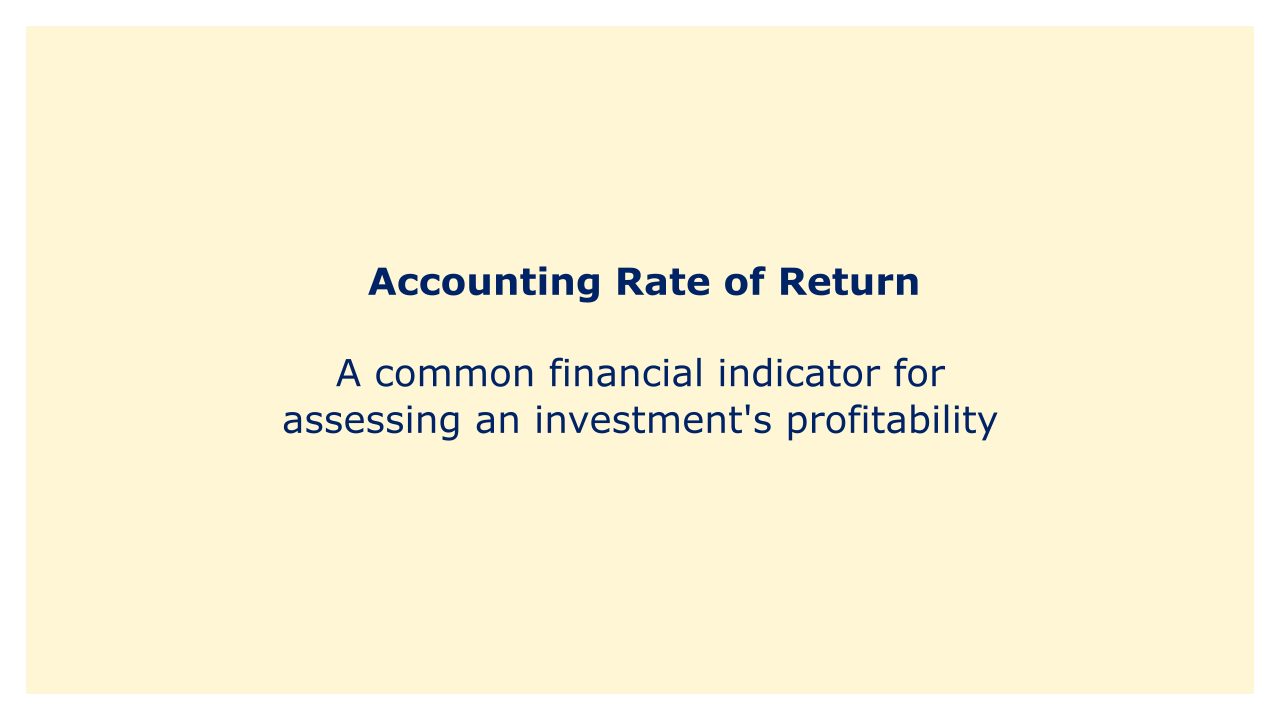# Accounting Rate of ReturnImage: Moneybestpal.com

### A common financial indicator for assessing an investment's profitability is the "accounting rate of return" (ARR). ARR is determined as the average annual return on investment over a given time period, usually stated as a percentage. The formula for ARR can be expressed as:

ARR = (Average Annual Profit / Average Investment) * 100

ARR is a metric used to quantify an investment's profitability in a clear-cut and uncomplicated manner. It is frequently applied in financial analysis, particularly when assessing capital expenditures and investment projects.

Consider the case of a business investing \$100,000 in a new project that is predicted to produce yearly earnings of \$20,000 for the following five years in order to demonstrate how ARR is calculated. The typical investment over the course of five years is \$100,000, and the typical annual return is \$20,000. The ARR for this investment would be:

ARR = (\$20,000 / \$100,000) * 100 = 20%

When assessing investment projects, it's important to take the ARR metric's limits into account. The ARR calculation, for instance, makes the assumption that the investment will be recovered at the end of the time period, which may not accurately reflect the investment's actual economic life. The time value of money, which can have a big impact on the profitability of an investment, is also not taken into account in the ARR calculation.

Tags